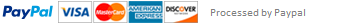### Computer Programming II Quadratic Equation Assignment

The purposes of this assignment include: passing of parameters, and command line parameters. You are to write a program to solve quadratic equations in all their possibilities (real or complex roots, or even degenerate equations). Your program should be invoked by the command:

where a, b, and c are coefficients. The solution should print on stdout.

If the solution is complex, the output should consist of a pair of expressions such as “2 + 3i” and “2 – 3i”. Other cases will result in either two real numbers being printed, a single real number, or perhaps just a message.

You must use separate compilation.

Main Program

• if 3 numbers provided on command line

◦ convert and assign coefficient a

◦ convert and assign coefficient b

◦ convert and assign coefficient c

◦ if a is zero

▪ if b is zero

• display error message (degenerate equation)

▪ otherwise

• solve linear equation (call solve_linear and send b,c)

◦ otherwise

• otherwise

◦ display error message (must provide 3 coefficients on command line)

solve_linear

• calculate x = - c / b

Hint: you may need to use a cast to handle integer division

• print x

• if b^2 – 4ac < 0

◦ solve for 2 complex solutions (call solve_complex and send a,b,c)

• otherwise

◦ solve for 2 real solutions (call solve_real and send a,b,c)

solve_real

• calculate x1

Hint: x1 = (-b + sqrt(b*b-4*a*c))/(2*a);

• calculate x2

• print x1 and x2

solve_complex

• calculate real part of solution

Hint: x_real = -b/2a

• calculate imaginary part of solution

Hint: x_imag= sqrt(b2 – 4ac)/2a

• print the real part and imaginary parts separated by a plus sign and followed by the letter i

Hint: printf(“%f + %fi\n”, x_real, x_imag);

• print the real part and imaginary parts separated by a minus sign and followed by the letter i

This assignment has been answered 6 times in private sessions.